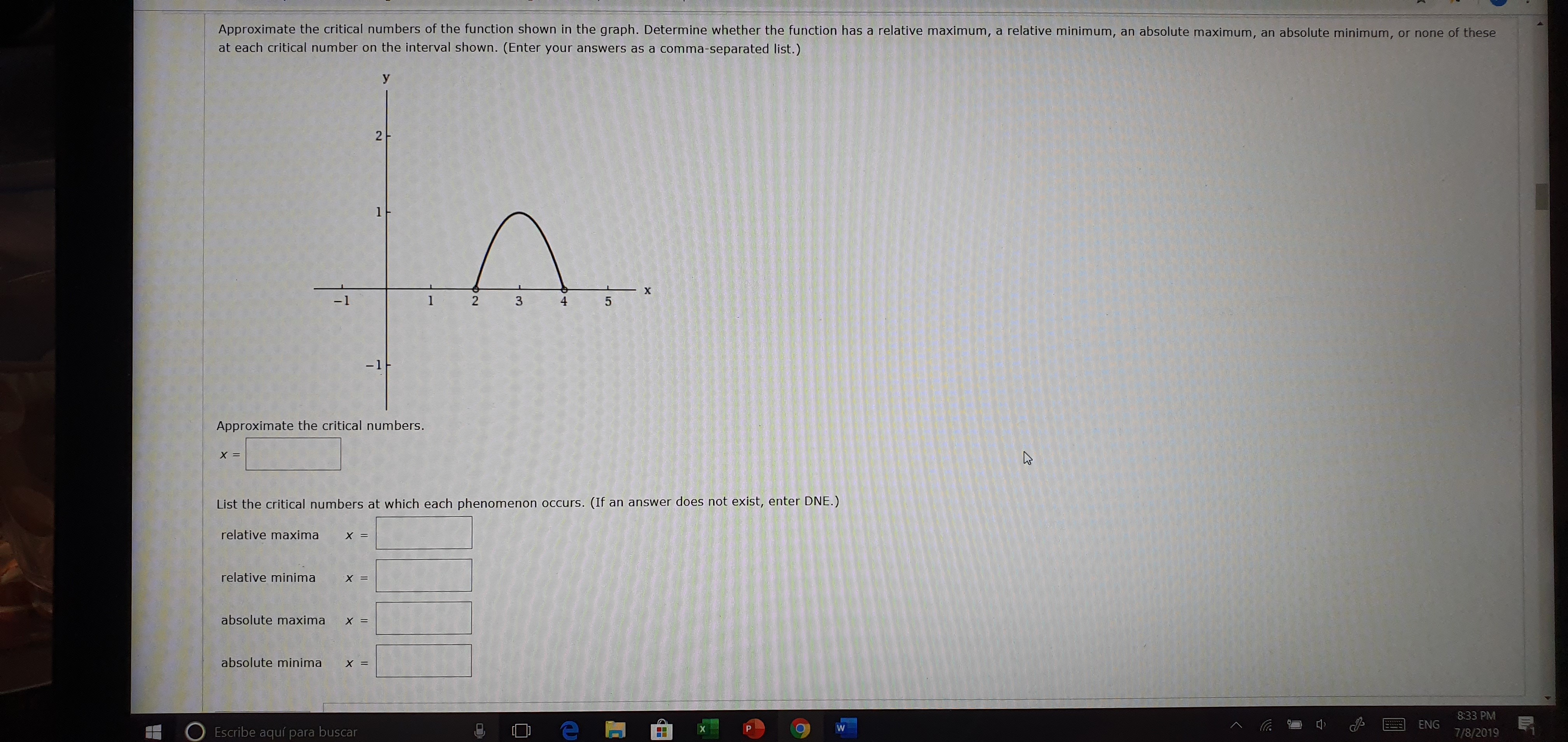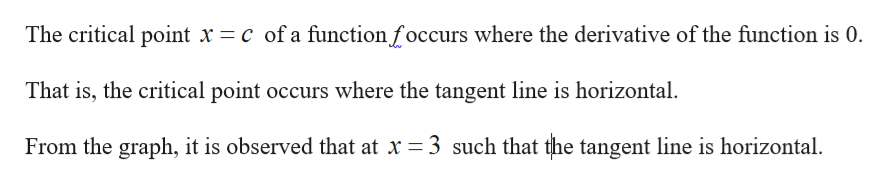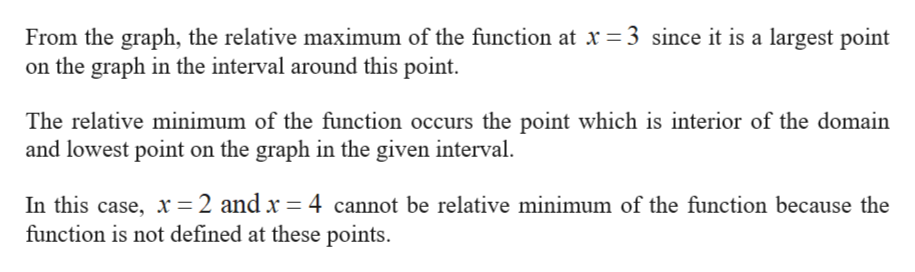# Approximate the critical numbers of the function shown in the graph. Determine whether the function has a relative maximum, a relative minimum,at each critical number on the interval shown. (Enter your answers as a comma-separated list.)an absolute maximum,an absolute minimum,or none of thesey21X-145-1FApproximate the critical numbers.X =(If an answer does not exist, enter DNE.)List the critical numbers at which each phenomenon occurs.relative maxima= Xrelative minima= Xabsolute maxima x=absolute minimaX =8:33 PMENGWEscribe aquí para buscar7/8/20192

Question
70 views

How can I get the result?

Which is the result?help_outlineImage TranscriptioncloseApproximate the critical numbers of the function shown in the graph. Determine whether the function has a relative maximum, a relative minimum, at each critical number on the interval shown. (Enter your answers as a comma-separated list.) an absolute maximum, an absolute minimum, or none of these y 2 1 X -1 4 5 -1F Approximate the critical numbers. X = (If an answer does not exist, enter DNE.) List the critical numbers at which each phenomenon occurs. relative maxima = X relative minima = X absolute maxima x= absolute minima X = 8:33 PM ENG W Escribe aquí para buscar 7/8/2019 2 fullscreen
check_circle

star
star
star
star
star
1 Rating
Step 1

Find the critical points.help_outlineImage TranscriptioncloseThe critical point x c of a functionfoccurs where the derivative of the function is 0. That is, the critical point occurs where the tangent line is horizontal From the graph, it is observed that at x = 3 such that the tangent line is horizontal fullscreen
Step 2

Find relative maxima and...help_outlineImage TranscriptioncloseFrom the graph, the relative maximum of the function at x = 3 since it is a largest point on the graph in the interval around this point The relative minimum of the function occurs the point which is interior of the domain and lowest point on the graph in the given interval. In this case, x= 2 and x = 4 cannot be relative minimum of the function because the function is not defined at these points fullscreen

### Want to see the full answer?

See Solution

#### Want to see this answer and more?

Solutions are written by subject experts who are available 24/7. Questions are typically answered within 1 hour.*

See Solution
*Response times may vary by subject and question.
Tagged in

### Calculus